Test: Circle- 2

# Test: Circle- 2

Test Description

## 10 Questions MCQ Test Mathematics for Class 5 | Test: Circle- 2

Test: Circle- 2 for Class 5 2022 is part of Mathematics for Class 5 preparation. The Test: Circle- 2 questions and answers have been prepared according to the Class 5 exam syllabus.The Test: Circle- 2 MCQs are made for Class 5 2022 Exam. Find important definitions, questions, notes, meanings, examples, exercises, MCQs and online tests for Test: Circle- 2 below.
Solutions of Test: Circle- 2 questions in English are available as part of our Mathematics for Class 5 for Class 5 & Test: Circle- 2 solutions in Hindi for Mathematics for Class 5 course. Download more important topics, notes, lectures and mock test series for Class 5 Exam by signing up for free. Attempt Test: Circle- 2 | 10 questions in 15 minutes | Mock test for Class 5 preparation | Free important questions MCQ to study Mathematics for Class 5 for Class 5 Exam | Download free PDF with solutions
 1 Crore+ students have signed up on EduRev. Have you?
Test: Circle- 2 - Question 1

### What is the radius of a circle whose diameter is 15 cm?

Detailed Solution for Test: Circle- 2 - Question 1

Using the formula:

d = 2 r

Solving for r: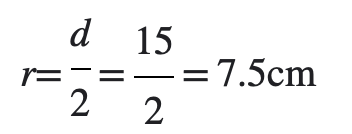Test: Circle- 2 - Question 2

### Is the black line a radius or diameter of the circle?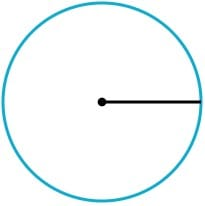Detailed Solution for Test: Circle- 2 - Question 2

The radius is half the length of the diameter and is joined to the centre at one end. Hence, it is the radius of the circle.

Test: Circle- 2 - Question 3

### Which segment is a radius?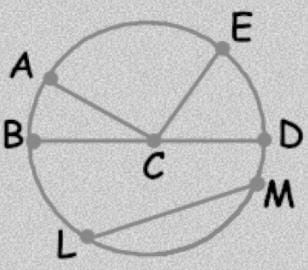Detailed Solution for Test: Circle- 2 - Question 3

The radius is half the length of the diameter and is joined to the centre at one end. Hence, DC is the radius of the circle.

Test: Circle- 2 - Question 4

Which of the following are the radii of two concentric circles?

Detailed Solution for Test: Circle- 2 - Question 4

If two or more circles that have the same center, but different radii they are termed as concentric circles.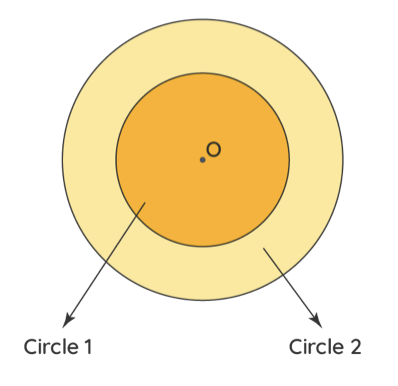Test: Circle- 2 - Question 5

What is the radius if the diameter is 12 m ?

Detailed Solution for Test: Circle- 2 - Question 5

Using the formula:

d = 2 r

Solving for r: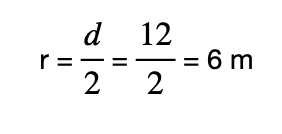Test: Circle- 2 - Question 6

Which is the correct definition of a circle?

Detailed Solution for Test: Circle- 2 - Question 6

A circle is the set of all points in a plane that are equidistant from a given point called the center of the circle.

Test: Circle- 2 - Question 7

What is the circumference of the given circle?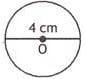Detailed Solution for Test: Circle- 2 - Question 7

Circumference of a circle whose radius is r is given by 2 π r

Here d= 4 cm

Hence, r is 2 cm

Circumference of the circle = 2 π (2)

Circumference of the circle = 4 π cm

Test: Circle- 2 - Question 8

Which of the following is a false statement?

Detailed Solution for Test: Circle- 2 - Question 8

A diameter of a circle is any straight line segment that passes through the center of the circle and whose endpoints lie on the circle.

Test: Circle- 2 - Question 9

_____________ is  a special chord that goes through the center of a circle.

Detailed Solution for Test: Circle- 2 - Question 9

A chord that passes through the center of a circle is called a diameter and is the longest chord of that specific circle.

Test: Circle- 2 - Question 10

What is the radius if the diameter is 40 m ?

Detailed Solution for Test: Circle- 2 - Question 10

Using the formula:

d = 2 r

Solving for r: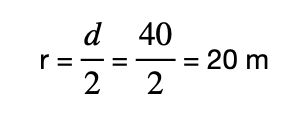## Mathematics for Class 5

10 videos|54 docs|35 tests
 Use Code STAYHOME200 and get INR 200 additional OFF Use Coupon Code
Information about Test: Circle- 2 Page
In this test you can find the Exam questions for Test: Circle- 2 solved & explained in the simplest way possible. Besides giving Questions and answers for Test: Circle- 2, EduRev gives you an ample number of Online tests for practice

## Mathematics for Class 5

10 videos|54 docs|35 tests